## Example Questions

### Example Question #1 : How To Find The Surface Area Of A Prism

If the dimensions of a rectangular crate are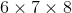, which of the following CANNOT be the total surface area of two sides of the crate?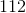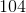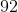Explanation:

Side 1: surface area of the 6 x 7 side is 42

Side 2: surface area of the 7 x 8 side is 56

Side 3: surface area of the 6 x 8 side is 48.

We can add sides 1 and 3 to get 90, so that's not the answer.

We can add sides 1 and 1 to get 84, so that's not the answer.

We can add sides 2 and 3 to get 104, so that's not the answer.

We can add sides 2 and 2 to get 112, so that's not the answer.

This leaves the answer of 92. Any combination of the three sides of the rectangular prism will not give us 92 as the total surface area.

### Example Question #2 : How To Find The Surface Area Of A Prism

Kate wants to paint a cylinder prism.

What is the surface area of her prism if it isinches tall and has a diameter ofinches? Round to the nearest whole number.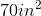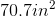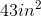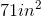Explanation:

First, find the area of the base of the cyclinder: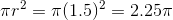and multiply that by two, since there are two sides with this measurement: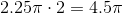Then, you find the width of the rectangular portion (label portion) of the prism by finding the circumference of the cylinder: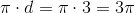. This is then multiplied by the height of the cylinder to find the area of the rectanuglar portion of the cylinder: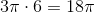.

Finally, add all sides together and round: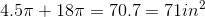.

### Example Question #3 : How To Find The Surface Area Of A Prism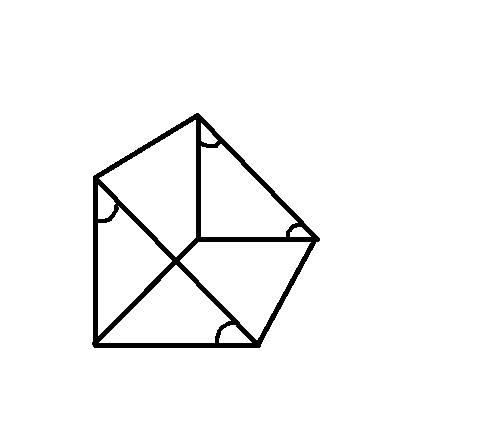This triangular prism has a height offeet and a length offeet.

What is the surface area of the prism? Round to the nearest tenth.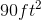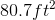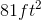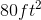Explanation:

Find the area of the triangular sides first: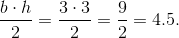Since there are two sides of this area, we multiply the area by 2: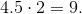Next find the area of the rectangular regions. Two of them have the width of 3 feet and a length of 7 feet, while the last one has a width measurement of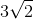feet and a length of 7 feet. Multiply and add all other sides: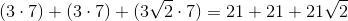.

Lastly, add the triangular sides to the rectangular sides and round: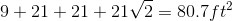.

### Example Question #1 : Prisms

A rectangular prism has a width of 3 units, a length that is twice the width, and a height that is two-thirds the length. What is the volume of the prism?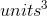Explanation:

Remember, the formula for the volume of a rectangular prism is width times height times length: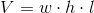Now, let's solve the word problem for each of these values. We know that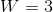. If length is double the width, then the length must be 6 units. If the height is two-thirds the length, then the height must be 4: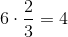Multiply all three values together to solve for the volume: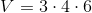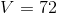The volume of the rectangular prism isunits cubed.

### Example Question #13 : Solid Geometry

The surface area of a rectangular prism is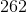, and the lengths of two sides areand. What is the volume of the prism?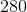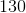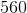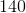Explanation:

The surface area of a rectangular prism with sides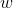, andis given as: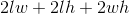Two sides are known; it does not matter how they are designated, but for this problem let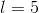and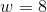, withas the unknown side. This yields equality: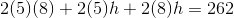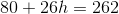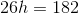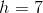Now that the three dimensions are known, it's possible to calculate the volume: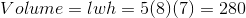Tired of practice problems?

Try live online GRE prep today.